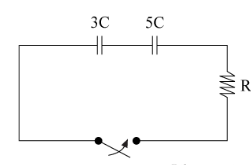# Problem: Two capacitors of capacitance 3C and 5C (where C = 0.13 F) are connected in series with a resistor of resistance R Randomized Variables R=5.5 Ω If the circuit was charged by a 10.0 V source how much total charge (in C) did both capacitors have in them to begin with?

###### FREE Expert Solution

Equivalent capacitance for two capacitors:

$\overline{){{\mathbf{C}}}_{\mathbf{e}\mathbf{q}}{\mathbf{=}}\frac{{\mathbf{C}}_{\mathbf{1}}{\mathbf{C}}_{\mathbf{2}}}{{\mathbf{C}}_{\mathbf{1}}\mathbf{+}{\mathbf{C}}_{\mathbf{2}}}}$

The charge stored in a capacitor:

$\overline{){\mathbf{Q}}{\mathbf{=}}{\mathbf{C}}{\mathbf{V}}}$

91% (332 ratings)###### Problem Details

Two capacitors of capacitance 3C and 5C (where C = 0.13 F) are connected in series with a resistor of resistance R Randomized Variables R=5.5 ΩIf the circuit was charged by a 10.0 V source how much total charge (in C) did both capacitors have in them to begin with?